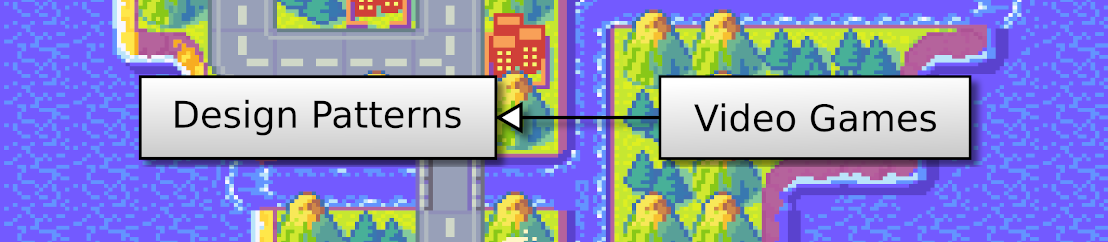## OpenGL 2D Facade (25): Get the Z of a pixel

In this post, I show how to get the z of a pixel using the OpenGL Z-Buffer. I use it to identify the tile below the mouse cursor. This approach is faster than ray casting, as it let the GPU do the job!

This post is part of the OpenGL 2D Facade series

## Objective

To check that it works fine, the player click on items in the world, and the character tells what it is:

## Get the Z of a pixel

### Ray casting

The usual approach is to cast a ray from the pixel and find the closest intersecting face. In 2D, we look for all the faces that contain the pixel. Since our faces are rectangles, the computation of the intersection is simple. On layers with regularity, like grids, it can be even easier. Once we found faces that contain the pixel, we read the tile texture to see if the pixel is transparent, in which case we ignore the face. In the end, we select the face with the lowest depth value.

As you can imagine, ray casting requires many computations. With the approach based on the Z-Buffer, we can reduce that do almost nothing and save CPU time for other tasks.

### From Z-Buffer to depth

We can ask OpenGL for any value of the Z-Buffer. For instance, we can get the Z-Buffer of a pixel (x,y):

``````data = glReadPixels(x, screenHeight - 1 - y, 1, 1, GL_DEPTH_COMPONENT, GL_FLOAT)
zbuffer = float(data)``````

Remind that the Y-axis of OpenGL is bottom-up, this is why we invert y.

This zbuffer value is in [0,1], so we need to convert it to NDC (Normalized Device Coordinates):

``z = 2 * zbuffer - 1``

Finally, we "linearize" this z value to get the depth of the pixel, as shown in the previous post:

``````zNear = 0.001
zFar = 1.0
maxDepth = 65536
a = maxDepth * zFar / (zFar - zNear)
b = maxDepth * zFar * zNear / (zNear - zFar)
depth = a + b / z``````

With these settings, the depth value is between 0 (front) and 65535 (background).

We extend the `ZBuffer` class with these formulae:

``````class ZBuffer:
zNear = 0.001
zFar = 1.0
maxDepth = 65536
a = maxDepth * zFar / (zFar - zNear)
b = maxDepth * zFar * zNear / (zNear - zFar)

@staticmethod
def depth2z(depth: float) -> float:
return ZBuffer.b / (depth - ZBuffer.a)

@staticmethod
def z2depth(z: float) -> float:
return ZBuffer.a + ZBuffer.b / z

@staticmethod
def zbuffer2z(zbuffer: float) -> float:
return 2 * zbuffer - 1

@staticmethod
def zbuffer2depth(zbuffer: float) -> float:
return ZBuffer.z2depth(2 * zbuffer - 1)``````

We also add a new method in the OpenGL facade that returns the depth of a pixel (x,y):

``````def getPixelDepth(self, x: int, y: int) -> float:
data = glReadPixels(x, self.screenHeight - 1 - y, 1, 1, GL_DEPTH_COMPONENT, GL_FLOAT)
zbuffer = float(data)
depth = ZBuffer.zbuffer2depth(zbuffer)
return depth``````

### From depth to layer

Since we assign a range of depth values for each layers, we can find the layer of a pixel. It is implemented in the `getPixelLayer()` method of the facade:

``````def getPixelLayer(self, x: int, y: int) ->
Tuple[Union[None, LayerGroup], int, Union[None, Layer], int]:
depth = int(round(self.getPixelDepth(x, y)))
for layerGroupIndex, layerGroup in enumerate(self.__layerGroups):
if layerGroup is None:
continue
for layerIndex, layer in enumerate(layerGroup):
if layer is None:
continue
if layer.hasDepth(depth):
return layerGroup, layerGroupIndex, layer, layerIndex
return None, -1, None, -1``````

Note the `hasDepth()` method of facade layers: it returns `True` if the layer uses the depth value, `False` otherwise. The implementation of these methods depends on each case and is straightforward.

### From grid layer to cell

Finding the face of a pixel depends on the type of the layer. In the case of a grid, we want the cell coordinates of the face. We add a new method `getPixelCell()` in the `GridLayer` class:

``````def getPixelCell(self, x: int, y: int) -> (int, int):
depth = int(round(self._gui.getPixelDepth(x, y)))
viewX, viewY = self._layerGroup.getTranslation()
cellX = (x + viewX) // self.tileWidth
for cellY, rowDepth in enumerate(self.__depths):
if rowDepth == depth:
return cellX, cellY
return -1, -1``````

Line 2 gets the depth of the pixel. We need it to find the right cell.

Line 3 gets the current shift of the layer. The coordinates of the pixel are relative to the screen or window; we need to translate them to world coordinates.

Line 4 translates the x screen/window coordinate to cell world coordinate. Note that we can't do the same with y coordinates because there are items larger than a row. For instance, big trees are two tiles tall.

Lines 5-7 parse all depths used by the layer and return the cell y coordinate corresponding to the pixel's depth.

### From characters layer to character indices

In the case of a characters layer, we want all the characters at some pixel location. We add a new method `getPixelCharacterIndices()` in the `CharactersLayer` class:

``````def getPixelCharacterIndices(self, x: int, y: int) -> List[int]:
depth = int(round(self._gui.getPixelDepth(x, y)))
if not self.hasDepth(depth):
return []
viewX, viewY = self._layerGroup.getTranslation()
return self.findFaces(x + viewX, y + viewY)``````

Lines 2-4 check that there is a character at screen/window coordinates (x, y). It can't be faster!

Line 5 gets the current shift of the layer to convert screen/window coordinates to world coordinates.

Line 6 uses a new method `findFaces()` of the `OpenGLLayer` class. It uses Numpy to find faces intersecting a given (faster than pure Python code):

``````def findFaces(self, x: float, y: float) -> List[int]:
spriteScreenX = -1 + x * self.__mesh.screenPixelWidth
spriteScreenY = 1 - y * self.__mesh.screenPixelHeight

x1 = self.__vertices[:, 1, 0]
y1 = self.__vertices[:, 1, 1]
x2 = self.__vertices[:, 3, 0]
y2 = self.__vertices[:, 3, 1]

mask = (x1 <= spriteScreenX <= x2) and (y2 <= spriteScreenY <= y1)